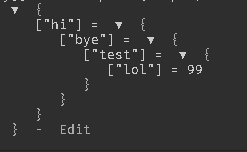# How can I make a table chain through loops?

Hey there, hope you are having a good day.
There seem to be a problem here!

I want to make a loop that creates table per table then sets a value for the last, for example:
This is the table i want to loop through

``````local array = {"hi","bye","test"}
local value = 99
``````

How can i make it so it will display like:

``````{
["hi"] = {
["bye"] = {
["test"] = 99
}
}
}
``````

Just in case if I add another value named `lol` then how can i make value go to `lol` instead of test?

``````local array = {"hi","bye","test","lol"}
local value = 99
``````

I want:

``````{
["hi"] = {
["bye"] = {
["test"] = {
["lol"] = 99
}
}
}
}
``````

I’ve been trying this for hours i’ve even tried to find but can’t find any solutions, (I know theres something related with maths, but i suck at maths!)
any help would be appreciated.

Regards.

``````local array = {"hi","bye","test","lol"}
local value = 99
local output = {}
local last = output
for i = 1, #array-1 do
last[array[i]] = {}
last = last[array[i]]
end
last[#array] = value
--return output
``````

Something like this (use output as your new table) should work. Can’t test the code so there may be minor mistakes. Abusing the fact that tables are stored by reference so that this should allow for chaining. Might be completely wrong.

2 Likes

For those who are looking for the same question
just replace

with

``````last[array[#array]] = value
``````Anyways it worked, tysm!!

1 Like